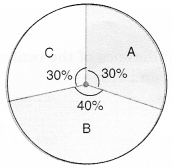• 108°
• 144°
• 72°
• 150°

• 108°
• 144°
• 72°
• 120°
• #### 3.  Observe the pie chart given below and answer the following questions:Which sector has the greatest angle?

• A
• B
• C
• None of these

• 36°
• 72°
• 81°
• #### 5.  Observe the pie chart and answer the following questions :Which two colours have the same central angles?

• Red, yellow
• Red, green
• Yellow, green
• Blue, red

• Red
• Yellow
• Green
• Blue
• #### 7.  Observe the pie chart and answer the following questions :The proportion of sector for red is

• $$\frac{1}{2}$$
• $$\frac{1}{4}$$
• $$\frac{1}{8}$$
• $$\frac{1}{3}$$
• #### 8.  Observe the pie chart and answer the following questions :The difference of the central angles for green and blue is

• 45°
• 90°
• 180°
• 22$$\frac{1°}{2}$$
• #### 9.  A child has a block in the shape of a cube with one letter written on each face as shown below:The cube is thrown once. What is the probability of getting A?

• $$\frac{1}{3}$$
• $$\frac{1}{6}$$
• $$\frac{1}{2}$$
• $$\frac{1}{4}$$
• #### 10.  A die is thrown. What is the probability of getting an even prime number?

• $$\frac{1}{6}$$
• $$\frac{1}{4}$$
• $$\frac{1}{3}$$
• $$\frac{1}{2}$$
Report Question
Practice More On Data Handling
access_time